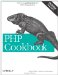# Recipe 25.4. Running PHP Code on Every Line of an Input File

#### 25.4.1. Problem

You want to read an entire file and execute PHP code on every line. For example, you wish to create a command-line version of grep that uses PHP's Perl-compatible regular expression engine.

#### 25.4.2. Solution

Use the -R command-line flag to process standard input:

`% php -R 'if (preg_match("/\$argv/", \$argn)) print "\$argn\n";'       php       < /usr/share/dict/words ephphatha`

To execute a block of code before or after processing the lines, use the -B and -E options, respectively:

`% php -B '\$count = 0;'       -R 'if (preg_match("/\$argv/", \$argn)) \$count++;'       -E 'print "\$count\n";'       php       < /usr/share/dict/words l`

#### 25.4.3. Discussion

Sometimes you want to quickly process a file using PHP via the command line, either as a standalone project or within a sequence of piped commands. This lets you whip up a quick-and-dirty script to transform data.

PHP makes that easy using three command-line flags and two special variables: -R, -B, -E, \$argn, and \$argi.

The -R flag specifies the PHP code you want to execute for every line in the file. Within that block of code, you can access the line's text in the \$argn variable.

As a basic example, here's a PHP script that takes HTML input, strips the tags, and prints out the result:

`php -R 'print strip_tags(\$argn) . "\n"; ' < index.html`

Since PHP automatically strips the newline from the end of the input, this code not only displays the results of strip_tags(\$argn), but also echos a newline.

It operates on the file index.html, which is passed in as standard input. There is no mechanism for specifying the file that you want processed.

This slightly more complicated example, which is a simple version of grep, shows how to accept input arguments via the \$argv array:

`% php -R 'if (preg_match("/\$argv/", \$argn)) print "\$argn\n";'       php       < /usr/share/dict/words ephphatha`

The first value passed preg_match( ) is /\$argv/, which is the first argument passed to the script. In this example, it's php, so this code is searching for all the words in the /usr/share/dict/words file containing php.

For what it's worth, ephphatha is an Aramaic word meaning "be opened."

Beyond the individual lines, you sometimes need to execute initialization or clean-up code. Specify this using the -B and -E flags.

Building on the grep example, this code counts the total number of matching lines:

`% php -B '\$count = 0;'       -R 'if (preg_match("/\$argv/", \$argn)) \$count++;'       -E 'print "\$count\n";'       php       < /usr/share/dict/words 1`

Inside the -B block, you initialize the \$count to 0. It's then incremented in the -R block whenever there's a match. Finally, the total number is printed out in the -E block.

To find out the percentage of matching lines, in addition to the total, use \$argi:

`% php -B '\$count = 0;'       -R 'if (preg_match("/\$argv/", \$argn)) \$count++;'       -E 'print "\$count/\$argi\n";'       php       < /usr/share/dict/words 1/234937`

The \$argi variable contains the current line number of the file, so inside the -E block, it's set to the total number of lines.

Documentation on Using PHP from the command line at http://www.php.net/features.commandline.PHP Cookbook: Solutions and Examples for PHP Programmers
ISBN: 0596101015
EAN: 2147483647
Year: 2006
Pages: 445

Similar book on Amazon